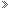Log in (Register | Forgotten password)You are not registered or have not logged in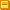Hello guest! (Not a guest? Log in above!) As a guest on TeenHelp you are only able to use some of our site's features. By registering an account you will be able to enjoy unlimited access to our site, and will be able to: Connect with thousands of teenagers worldwide by actively taking part in our Support Forums and Chat Room. Find others with similar interests in our Social Groups. Express yourself through our Blogs, Picture Albums and User Profiles. And much much more! Signing up is free, anonymous and will only take a few moments, so click here to register now!

Education and Careers Work of any kind can get stressful at times. Ask in this forum if you need help with coursework, applications, and more.

 Christinaa317o8 Offline Christina Regular TeenHelper*****   Name: Christina Age: 27 Gender: Female Location: New York Posts: 366 Blog Entries: 1 Join Date: January 29th 2009 Geometry Homework Help? - May 28th 2009, 02:09 PM So I stayed home from school today because I owe two assignments and I really need to get them done. I can't ask my teacher for help on them because she'll say its not her job to teach me 9th grade material that I forgot. I forgot how to solve systems of equations and I don't have a graphing calculator. The directions are to solve every system of equation graphically and algerbraically. If someone could explain how to do it I might be able to understand. I googled it and I couldn't find anything helpful. To show our work, we have the table with the three colums with x on the left, equation in the middle and y on the end. Here's number 1: y = x^2 - 4 y + x^2 = 4 Thanks to anyone who helps.Love is all we need. :]FORMER [HelpLinkMentor][LiveHelpOperator]
 Thurineth Offline Member I've been here a while********   Name: Laura Age: 30 Gender: Female Location: Aberdeen Posts: 1,140 Join Date: May 28th 2009 Re: Geometry Homework Help? - May 28th 2009, 02:41 PM I'm unsure what you mean? Is x and y the same in both the equations you gave?
 Christinaa317o8 Offline Christina Regular TeenHelper*****   Name: Christina Age: 27 Gender: Female Location: New York Posts: 366 Blog Entries: 1 Join Date: January 29th 2009 Re: Geometry Homework Help? - May 28th 2009, 02:52 PM I don't know. =[ Thats all that it says, with a graph next to it. I know that they're suppossed to be parabolas. the second problem is: y = x^2 - 5x + 8 y = -x^2 + 7x - 10Love is all we need. :]FORMER [HelpLinkMentor][LiveHelpOperator]
 <:3 )~ Offline squeak Average Joe***   Name: olivia Age: 28 Gender: Female Location: Across the Universe Posts: 195 Blog Entries: 2 Join Date: May 26th 2009 Re: Geometry Homework Help? - May 28th 2009, 02:54 PM This is a system of equations. Yippee :P y = x^2 - 4 re-arrange the second equation: y + x^2 = 4 y=-x^2+4 Go to Y=x^2-4 Down button, y=-x^2+4 Graph (upper right hand, under screen) Also, make sure all of your plots above are not darkened. Now, the interesection of both lines is the solution to the system. Go to graph. 2nd->Trace->5 Curve 1?: enter Curve 2?: enter x=2 and x=-2 for this system. y = x^2 - 5x + 8 y = -x^2 + 7x - 10 This one I would use the graphing calculator for. Go to Y=x^2-5x+8 Down button, y=-x^2+7x-10 Graph (upper right hand, under screen) Also, make sure all of your plots above are not darkened. Now, the interesection of both lines is the solution to the system. Go to graph. 2nd->Trace->5 Curve 1?: enter Curve 2?: enter x=2.9999992 which really just means x=3 for this system. (the reason being that it rounds up due to those tricky pixels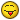Last edited by <:3 )~; May 28th 2009 at 03:05 PM.
 Thurineth Offline Member I've been here a while********   Name: Laura Age: 30 Gender: Female Location: Aberdeen Posts: 1,140 Join Date: May 28th 2009 Re: Geometry Homework Help? - May 28th 2009, 02:54 PM Right, I'm presuming the intercections have to go into "x" and "Ya columns? Are you able to give more info on whats to be done?
 Christinaa317o8 Offline Christina Regular TeenHelper*****   Name: Christina Age: 27 Gender: Female Location: New York Posts: 366 Blog Entries: 1 Join Date: January 29th 2009 Re: Geometry Homework Help? - May 28th 2009, 03:40 PM I don't have a graphing calculator though. I'm lost on how to do it by hand. We did this one in class xy = -6 x + 3y = 3 the table looked like this: x l xy = 6 l y -6 -3 -2 -1 1 2 3 6 I forget how to get the middle column. :/ and the y column is the same as the "X" column but in reverse order.Love is all we need. :]FORMER [HelpLinkMentor][LiveHelpOperator]
 pbandjay Offline Member Welcome me, I'm new!*   Name: Joseph Age: 33 Gender: Male Location: Iowa, US Posts: 24 Join Date: March 29th 2009 Re: Geometry Homework Help? - May 28th 2009, 06:49 PM For the first one, I will do it algebraically first. You have: y = x^2 - 4 and y + x^2 = 4. If you like, rearrange the second one so the terms match: y = x^2 - 4 y = -x^2 + 4 You are looking for x and y values that satisfy both of these equations. We know that y is explicitly equal to both x^2 - 4 and -x^2 + 4 so we can set both of these equal and then solve for x: x^2 - 4 = -x^2 + 4 (and then we can use our algebra skills) 2x^2 = 8 x^2 = 4 x = -2 and 2 Now we plug -2 in for x in one of the original equations to check which y-value corresponds to the solution with this x-value, so: y = (-2)^2 - 4 = 0, so the solution is x=-2 and y=0, or (-2,0). But wait, we have another x-value: 2. We plug this into one of the above equations (it doesn't matter which one) and still get y=0, so our second solution is (2,0). We have solutions: (-2,0) and (2,0). If we want to do this geometrically, the values of x and y that satisfy both of these equations are the same as the x and y values that the graphs of these functions share (where they overlap). If we graph y = x^2 - 4, we have the graph of parabola y=x^2, but moved downward four values. If you don't know how to graph this function, then use an x-y chart and pick x-values, such as 0,1,-1,2,etc... and find the y-values, then graph these points and connect the dots. If we graph y = -x^2 + 4 we have an upside down parabola y=x^2 but moved upward four values. And we see that these graphs overlap at (2,0) and (-2,0), which is the same as our algebraic solutions. For the second equation, let us do it algebraically first. We know: y = x^2 - 5x + 8 and y = -x^2 + 7x - 10, and we are looking for the values of x and y that satisfy both of these equations. Like before, we know that y equals both of the right sides, so we can plug one of the right sides in for y in the other equation (or set both expressions equal to each other), and we get: x^2 - 5x + 8 = -x^2 + 7x - 10 (move everything to one side) 2x^2 - 12x + 18 = 0 (we can divide by 2 and not change the equality) x^2 - 6x + 9 = 0 (now we have to experiment and factor) (x - 3)(x - 3) = 0 (x - 3)^2 = 0 If we set x = 3 in that last line, we will get 0 = 0 so we know that x=3 is a solution and the only solution since no other x-values turn the left side into 0. (The squared part does say something about the originally functions' behavior but it's not necessary to get into that I don't think.) Now we need to find the y-value that corresponds to this x-value in solving both of the equations. (It doesn't matter which originally equation we use.) So let's plug x=3 into one of them: y = 3^3 - 5(3) + 8 = 2 So our solution is (3,2). To find this solution geometrically: Graphing these equations without a graphing calculator is pretty easy but requires "completing the square" to change the equations into forms which tell you the exact x- and y-intercepts and such. After all of this typing it seems like it would just be too much heh. But if we graph both of these equations on the graphing calculator we see that there is one parabola suspended where its vertex is in the 1st quadrant and another upside down parabola with vertex in the 1st quadrant, and they both touch at (3,2) and only at that point. If any of this is confusing let me know, haven't had much sleep in the past two days..
 InSovietRussiaORGASMGotU Offline Member I can't get enough*********Posts: 2,088 Join Date: January 6th 2009 Re: Geometry Homework Help? - May 28th 2009, 07:44 PM The table you're describing seems to be a table of values, although I do it in a slightly simpler way. As you know, to graph you need values in the form of (x,y). The table of values generally has x on the left and in the other column, it has y = [equation]. This allows you to get the values as (x,y). y = x^2 - 4 1 y + x^2 = 4 2 It's much easier to make the equation have only 1 variable. I labelled them 1 and 2 just so it's simpler for me to write. Sub 1 into 2: x^2-4 + x^2 = 4 2x^2 = 8 x^2 = 4 x = +/-2 Since 1 has x^2, we'll get the same y-value regardless if x = -2 or x = 2: Sub x = 2 into 1: y = 2^2-4 y = 0 Therefore, solution of (2,0) and (-2,0). For the table format, choose whatever x-values you want and plug them into 1. y = x^2 - 5x + 8 1 y = -x^2 + 7x - 10 2 In 1 and 2, we see both have y = blah blah and y = blah blah. So, make it y = y: x^2-5x+8 = -x^2+7x-10 2x^2-12x + 18 = 0 We see that we can factor out a 2 to make it simpler: 2(x^2-6x+9) = 0 x^2-6x+9 = 0 We can factor this out through common factoring, Quadratic Equation, graphing calculator, whatever one you really want. The basic idea though is choose 2 numbers, and see if they add to the middle one (-6) and if they multiply to give the third one (9). We can choose either 1x9 or 3x3 (or decimals or whatever but common sense tells us we won't need that). 1 and 9 cannot give us a -6. -3+(-3) can give us -6. -3x-3 = 9 So, x^2-6x+9 = 0 factors to (x-3)(x-3) = 0 or simply (x-3)^2 = 0. To get any y-values, put the x-values into 1 or 2. I believe they'll give you the same answer, so pick which one you want. For both of these questions, you can graph the equations by hand, although you'll need to do more calculations, which I assume you're not doing right now.

 Tags geometry, homeworkThread Tools Search this Thread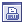Show Printable Version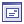Email this Page Search this Thread: Advanced Search Rate This Thread Rate This Thread: 5 : Excellent 4 : Good 3 : Average 2 : Bad 1 : TerriblePosting Rules You may post new threads You may post replies You may not post attachments You may not edit your posts BB code is On Smilies are On [IMG] code is On HTML code is OffTrackbacks are Off Pingbacks are Off Refbacks are Off Forum Rules